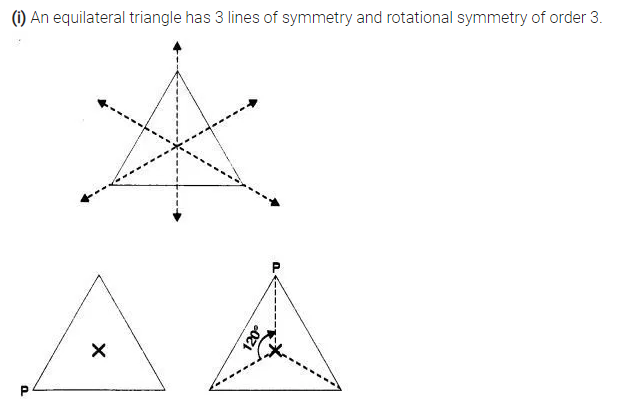# Draw, wherever possible, a rough sketch of (i) a triangle with both line and rotational symmetry of order more than 1

Draw, wherever possible, a rough sketch of
(i) a triangle with both line and rotational symmetry of order more than 1.
(ii) a triangle with only line symmetry and no rotational symmetry of order more than 1.
(iii) a quadrilateral with a rotational symmetry of order more than 1 but not a line symmetry.
(iv) a quadrilateral with line symmetry but not a rotational symmetry of order more than 1.